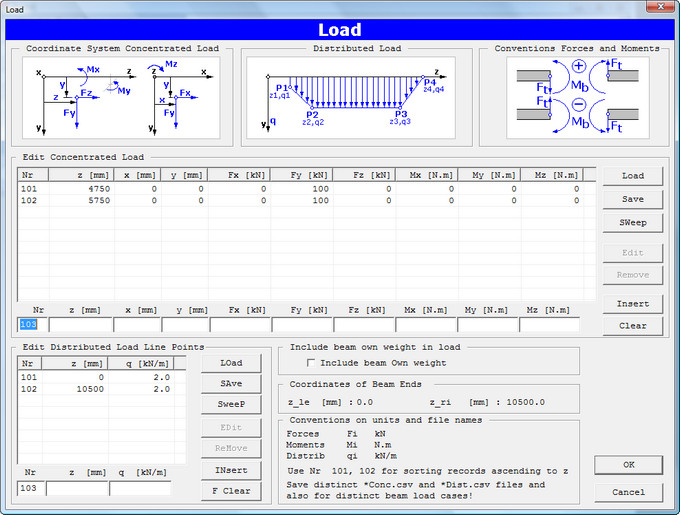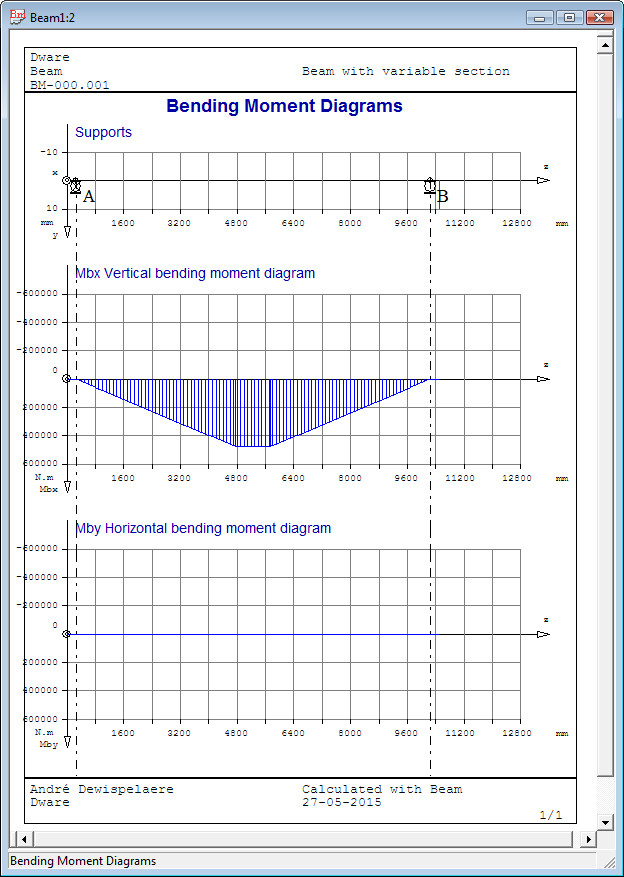### Calculation of beam weight

Calculation of beam weight and the location of centroid.

### Calculation of section properties

For each section at interval Dz the section properties are calculated: coordinates of centroid xG and yG, cross-sectional area A [mm²], moment of inertia Ix, Iy [mm4] and torsional moment of inertia It [mm4].

Input of beam load with dialog box:Input of concentrated load: the load is to define with coordinates of action point z, x, y [mm], forces Fx,y,z [kN] and moments Mx,y,z [N.m]. The coordinates x and y offer the possibility to define excentric loads. Input of distributed load by input of load line points z, q [kN/m]. The distributed load is converted to a discrete load acting in the centroid of the area under the load line. The user can check off if the own weight of the beam is to add to the load list. This option is disabled if the shaft cross section profile has not been defined, e.g. in the dimensioning problem.
The distributed load is described as a distirubuted force load caused by a mass rigidly connected to the beam and for the calculation of the natural frequency this force load is converted to mass.

### Calculation of Reaction Forces in Supports

The reaction forces in supports A, B, C ... are determined in form Rx,y,z [kN]. For a cantilever beam the reaction moment Mx,y,z [N.m] is calculated.

### Calculation of Bending Moment Diagram

For each cross section in location coordinate z on distance Dz from each other are calculated: bending moments Mbx, Mby and torsional moment Mz [N.m] and axial force Fz [kN] if present.
The calculation records are displayed on the screen in a table. Graphical representation in a bending moment diagram, as in figure underneath:### Calculation of Transverse Forces

For each calculation point of the moment diagram, the transverse forces Tx, Ty [kN] and normal force Fz [kN] are calculated. Graphic representation in a transverse force diagram.

Go to next web page for stress and deflection calculation.